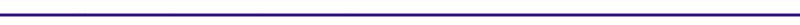StoichiometryIntroduction

Typical questions that this section on combustion addresses are:

What fuels are used for combustion ?

What are the typical combustion products ?

How many moles of each combustion product are produced during a combustion reaction ?

Air and fuel mix in the piston cylinder to form the reactants of combustion. If we assume all the fuel will be consumed during the reaction, at temperatures less than 1000 K, the chemical reaction can written as: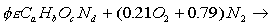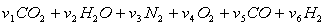Where: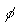= equivalence ratio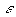= the molar fuel/air ratio
a,b,c,d = fuel components
v's = moles of product per mole of air

Equilibrium Combustion Products

If the final product temperatures less than 1000 K, the products are CO2, H2O, N2,O2, CO, and H2, with exact concentrations depending on the reaction temperature and fuel/air ratio. At high fuel/air ratios, there is insufficient oxygen to convert all of the carbon in the fuel to carbon dioxide, resulting in the formation of carbon monoxide.

At temperatures greater than 1000 K, dissociation reactions during the combustion process will result in additional species, including OH, NO, H and O. In an internal combustion engine, NO is formed during the period of combustion when the reaction temperatures are above 2000 K. The decomposition rate of NO is very slow at temperatures below 2000 K, so the NO concentrations "freeze" at concentrations greater than the equilibrium values at lower temperatures.

Definitions

Fuel/Air Ratio

The molar fuel/air ratio is (for 21% oxygen and 79% nitrogen):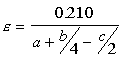Equivalence Ratio

The equivalence ratio is defined as the ratio of the actual fuel/air ratio to the stoichiometric fuel/air ratio. Stoichiometric combustion occurs when all the oxygen is consumed in the reaction, and there is no molecular oxygen(O2) in the products.

If the equivalence ratio is equal to one, the combustion is stoichiometric. If it is < 1, the combustion is lean with excess air, and if it is >1, the combustion is rich with incomplete combustion.

Fuels

Six common fuels are:

CH4      Methane            CH3OH      Methanol

C3H8     Propane            C2H5OH    Ethanol

C8H18   Octane              H2              Hydrogen

Low Temperature (T< 1000 K)Solution for the determination of the combustion products

The solution of for the moles of CO per moles of air, v5, is given by: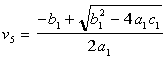Where:
a1 = 1-K
b1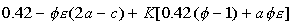c1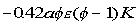K =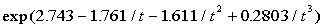t = T/1000
T = temperature of combustion [K]

Based on the equivalence ratio the solutions for the molar coefficients of the products are:

Table I. Solutions for the molar coefficents of the products

 Products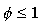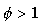CO2 v1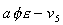H2O v2N2 v3O2 v40 CO v5 0 v5 H2 v6 0